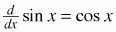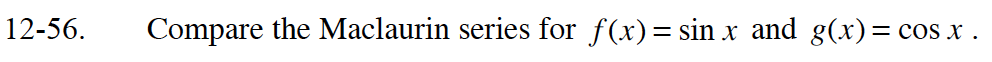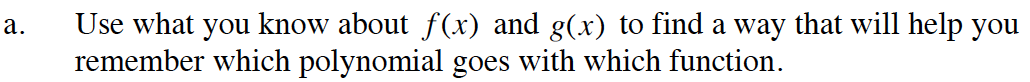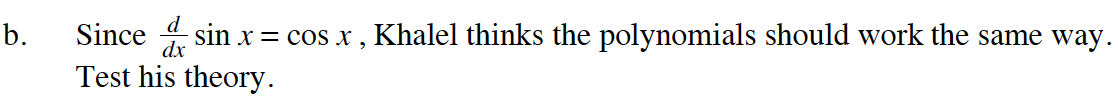### Home > CALC > Chapter 12 > Lesson 12.1.5 > Problem12-56

12-56.
1. Compare the Maclaurin series for f(x) = sin x and g(x) = cos x . Homework Help ✎

1. Use what you know about f(x) and g(x) to find a way that will help you remember which polynomial goes with which function.

2. Since, Khalel thinks the polynomials should work the same way. Test his theory.$\sin(x)=x-\frac{x^3}{3!}+\frac{x^5}{5!}-\frac{x^7}{7!}+...$